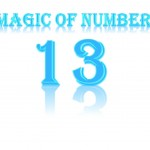# Magic of number 13Every one used to say that 13 is an unlucky number or devil number. But for Dr.kaprekar, 13 looks like a wonderful number. He identified many specialties in number 13 and made a big list.

13 is one of the number in twin prime number. In this you can see first to prime number comes next by next. The difference between the two numbers is 2 and the sum of two number is 2 square.

3 – 1 = 2
3 + 1 = 22

The square of no. 13 is 169.The same number you write from right to left you will get 961. This 961 is the answer of 312,you just write number 13 from right to left you will get 31.

13 x 13 = 169
31 x 31 = 961

169 you just separate it as 16 and 9.
16 is the answer of 42 and 9 is the answer of 32.
Now you just add 16 + 9 = 25. 25 is the answer of 52.
Now you multiply 16 x 9 = 144. It is an answer of 122.

The number 13 you can show it from 3 individual numbers also. write 3 indidual number togeather and square it you will get 6 digit answer, you separate that 6 digit number in to 3 part and add it that will be the answer of 13 square.

Number 13        =  8 + 2 + 3;     = 8232 = 677329

67 + 73 + 29 = 169 = 132

=  3 + 2 + 8      =3282 = 107584

10 + 75 + 84 = 169 = 132

= 9 + 2 + 2 = 9222 =850084

85 + 00 + 84 = 132

By,
Rushalli

1.2.